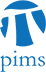## SCAIM Seminar: Preconditioning Iterative Methods for the Optimal Control of the Stokes Equations

• Date: 06/08/2010
Location:

University of British Columbia

Description:

Solving problems regarding the optimal control of partial differential equations (PDEs) - a type of PDE-constrained optimization - is a frontier area of numerical analysis. Of particular interest is the problem of flow control, where one would like to effect some desired flow by exerting, for example, an external force. The bottleneck in many current algorithms is the solution of the optimality system - a system of equations in saddle point form that is usually very large and ill-conditioned. In this talk I will describe a block-diagonal preconditioner for the minimal residual method which can be applied to such problems where the PDEs are the Stokes equations. We will consider only distributed control here, although other problems - for example boundary control - can be solved in a similar way. I will present numerical results, and compare these with those obtained by solving the
equivalent forward problem using similar techniques.

Schedule:

12:30 - 14:00, WMAX 216.

Pizza and pop will be provided!

Other Information:

For details, please visit the official website at